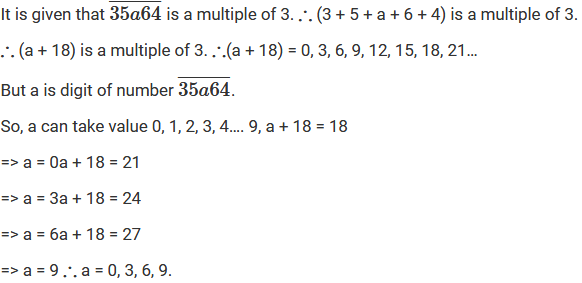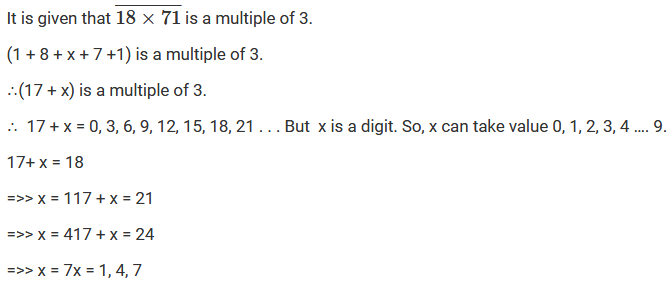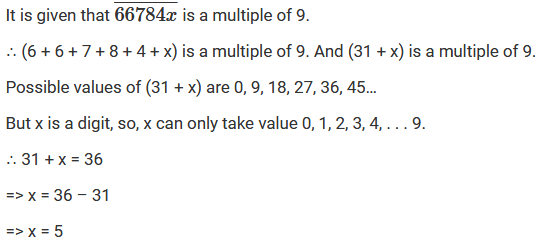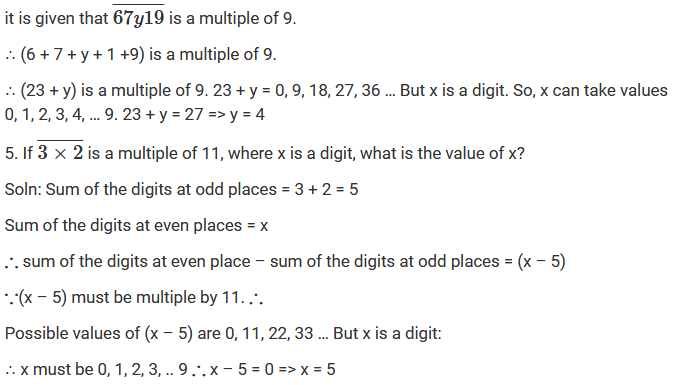×#### Thank you for registering.

One of our academic counsellors will contact you within 1 working day.

Click to Chat

1800-1023-196

+91-120-4616500

CART 0

• 0

MY CART (5)

Use Coupon: CART20 and get 20% off on all online Study Material

ITEM
DETAILS
MRP
DISCOUNT
FINAL PRICE
Total Price: Rs.

There are no items in this cart.
Continue Shopping• Complete JEE Main/Advanced Course and Test Series
• OFFERED PRICE: Rs. 15,900
• View Details

Chapter 5: Playing With Numbers Exercise – 5.2

Question: 1

Given that the number is divisible by 3, where a is a digit, what are the possible values of a.

Solution:Question: 2

If x is a digit of the number is divisible by 3, find possible values of x.

Solution:Question: 3

If x is a digit of the number  such that it is divisible by 9, find possible values of x.

Solution:Question: 4

Given that the number is divisible by 9, where y is a digit, what are the possible values of y?

Solution:Question: 5

If  is a multiple of 11, where x is a digit, what is the value of x?

Solution:

Sum of the digits at odd places = 3 + 2 = 5

Sum of the digits at even places = xQuestion: 6

If  is a number with x as its tens digit such that it is divisible by 4. Find all possible values of x.

Solution:

A natural number is divisible by 4 if the number formed by its digits in units and tens places in divisible by 4.Question: 7

If x denotes the digit at hundreds place of the number  such that the number is divisible by 11. Find all possible values of x

Solution:

A number is divisible by 11, if the difference of the sum of its digits at odd places and the sum of its digits at even places is either 0 or a multiple of 11.

Sum of digits at odd places – sum of digits at even places – (6 + x + 9) – (7 + 1) = (15 + x) – 8 = x + 7

∴ x + 7 = 11 => x = 4

Question: 8

Find the remainder when 981547 is divided by 5. Do this without doing actual division.

Solution:

If a natural number is divided by 5, it has the same remainder when its unit digit is divided by 5.

Here, the unit digit of 981547 is 7. When 7 is divided by 5, remainder is 2.

Therefore, remainder will be 2 when 981547 is divided by 5.

Question: 9

Find the remainder when 51439786 is divided by 3. Do this without performing actual division.

Solution:

Sum of the digits of the number 51439786 = 5 + 1 + 4 + 3 + 9 + 7 + 8 + 6 = 43.

The remainder of 51439786, when divided by 3, is the same as the remainder when the sum of the digits is divided by 3.

When 43 is divided by 3, the remainder is 1.

Therefore, when 51439786 is divided by 3, the remainder will be 1.

Question: 10

Find the remainder, without performing actual division, when 798 is divided by.

Solution:

798 = A multiple of 11 + (sum of its digits at odd places – sum of its digits at even places) 798

= A multiple of 11 + (7 + 8 – 9) 798

= A multiple of 11 + (15 – 9)798

= A multiple of 11 + 6

Therefore, the remainder is 6.

Question: 11

Without performing actual division, find the remainder when 928174653 is divided by 11.

Solution:

928174653 = A multiple of 11 + (Sum of its digits at odd places – sum if its digits at even places) 928174653

= A multiple of 11 + {(9 + 8 + 7 + 6 + 3) – (2 + 1 + 4 + 5)} 928174653

= A multiple of 11 + (33 – 12)928174653

= A multiple of 11 + 21928174653

= A multiple of 11 + (11 x 1 + 10)928174653

= A multiple of 11 + 10.

Therefore, the remainder is 10.

Question: 12

Given an example of a number which is divisible by:

(i) 2 but not by 4

(ii) 3 but not by 6

(iii) 4 but not by 8

(iv) both 4 and 8 but not by 32.

Solution:

(i) 10 Every number with the structure (4n + 2) is an example of a number that is divisible by 2 but not by 4.

(ii) 15 Every number with the structure (6n + 3) is an example of a number that is divisible by 3 but not by 6.

(iii) 28 Every number with the structure (8n + 4) is an example of a number that is divisible by 4 but not by 8.

(iv) 8 Every number with the structure (32n + 8), (32n + 16) or (32n + 24) is an example of a number that is divisible by 4 and 8 but not by 32.

Question: 13

Which of the following statements are true?

(i) If a number is divided by 3, it must be divisible by 9.

(ii) If a number is divisible by 9, it must be divisible by 3.

(iii) If a number is divisible by 4, it must be divisible by 8.

(iv) If a number is divisible by 8, it must be divisible by 4

(v) A number is divisible by 18, If it is divisible by both 3 and 6.

(vi) If a number is divisible by both 9 and 10, it must be divisible by 90

(vii) If a number exactly divides the sum of two numbers, it must exactly divides the numbers separately.

(viii) If a number divides three numbers exactly, it must divide their sum exactly.

(ix) if two numbers are co-prime, at least one of them must be a prime number.

(x) The sum of two consecutive odd numbers is always divisible by 4

Solution:

(i) False

Every number with the structure (9n + 3) or (9n + 6) is divisible by 3 but not by 9.

(ii) True

(iii) False

Every number with the structure (8n + 4) is divisible by 4 but not by 8.

(iv) True

(v) False

(vi) True

(vii) False

(viii) True

(ix) False

(x) True### Course Features

• 728 Video Lectures
• Revision Notes
• Previous Year Papers
• Mind Map
• Study Planner
• NCERT Solutions
• Discussion Forum
• Test paper with Video Solution